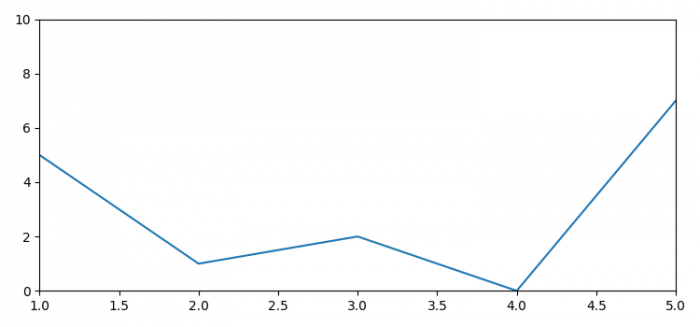# How to remove whitespaces at the bottom of a Matplotlib graph?

To remove whitespaces at the bottom of a Matplotlib graph, we can use tight layout or autoscale_on=False.

## steps

• Set the figure size and adjust the padding between and around the subplots.

• Create a new figure or activate an existing figure.

• Add an 'ax' to the figure as part of a subplot arrangement.

• Plot a list of data points using plot() method.

• To display the figure, use show() method.

## Example

from matplotlib import pyplot as plt

plt.rcParams["figure.figsize"] = [7.50, 3.50]
plt.rcParams["figure.autolayout"] = True

fig = plt.figure()

ax = fig.add_subplot(111, autoscale_on=False, xlim=(1, 5), ylim=(0, 10))
ax.plot([2, 5, 1, 2, 0, 7])

plt.show()

## Output